Engineering Mechanics - 2

# Engineering Mechanics - 2

Test Description

## 20 Questions MCQ Test Mechanical Engineering SSC JE (Technical) | Engineering Mechanics - 2

Engineering Mechanics - 2 for Mechanical Engineering 2023 is part of Mechanical Engineering SSC JE (Technical) preparation. The Engineering Mechanics - 2 questions and answers have been prepared according to the Mechanical Engineering exam syllabus.The Engineering Mechanics - 2 MCQs are made for Mechanical Engineering 2023 Exam. Find important definitions, questions, notes, meanings, examples, exercises, MCQs and online tests for Engineering Mechanics - 2 below.
Solutions of Engineering Mechanics - 2 questions in English are available as part of our Mechanical Engineering SSC JE (Technical) for Mechanical Engineering & Engineering Mechanics - 2 solutions in Hindi for Mechanical Engineering SSC JE (Technical) course. Download more important topics, notes, lectures and mock test series for Mechanical Engineering Exam by signing up for free. Attempt Engineering Mechanics - 2 | 20 questions in 12 minutes | Mock test for Mechanical Engineering preparation | Free important questions MCQ to study Mechanical Engineering SSC JE (Technical) for Mechanical Engineering Exam | Download free PDF with solutions
 1 Crore+ students have signed up on EduRev. Have you?
Engineering Mechanics - 2 - Question 1

### On a ladder resting on a smooth ground and leaning against vertical wall, the force of friction will be:

Detailed Solution for Engineering Mechanics - 2 - Question 1

Free body diagram of a ladder resting on a frictional surface is: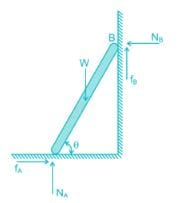The force of friction will be upwards at its upper end and towards the wall at its lower end.

As the ground is smooth (but the wall does not), so fA = 0 and fB ≠ 0

So the force of friction will be upwards at its upper end.

Engineering Mechanics - 2 - Question 2

### A body moves with a speed of 10 m/s in the curved path of 25 m radius of curvature. If the tangential acceleration is 3 m/s2, then total acceleration for the body will be:

Detailed Solution for Engineering Mechanics - 2 - Question 2

Normal component: an = v2/r = (10)2/(25) = 4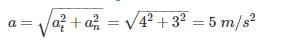Engineering Mechanics - 2 - Question 3

### A person who weighs 800 N steps onto a scale that is on the floor of an elevator car. If the elevator accelerates upward at a rate of 5 m/s2, what will the scale read?

Detailed Solution for Engineering Mechanics - 2 - Question 3

The person exerts a downward force on the scale, and the scale pushes up on the person with an equal (but opposite) force, FN. Thus, the scale reading is FN, the magnitude of the normal force.

FN – FW = ma ⇒ FN – mg = ma

⇒ F= ma + mg = m (a + g) = (W/g) (a + g) = (800/10) (10 + 5)

F= 1200 N

Engineering Mechanics - 2 - Question 4

Two forces of 30 N and 40 N are acting at 90° to each other, the resultant force is

Detailed Solution for Engineering Mechanics - 2 - Question 4

Resultant between two forces P and Q, acting at an angle θ is defined as: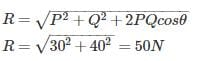Engineering Mechanics - 2 - Question 5

A fan consumes 20 W of electric power and discharges air from a ventilated room at 0.25 kg/s. The maximum air outlet velocity is nearly

Detailed Solution for Engineering Mechanics - 2 - Question 5

A fan is claimed to increase the velocity of air to a specified value while consuming electric power at a specified rate. The electric power input will be equal to the rate of increase of the kinetic energy of air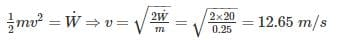Engineering Mechanics - 2 - Question 6

Which of the following forces have zero value of resultant?

Detailed Solution for Engineering Mechanics - 2 - Question 6

Equilibrium is the status of the body when it is subjected to a system of forces. If the resultant force is equal to zero it implies that the net effect of the system of forces is zero this represents the state of equilibrium.

Engineering Mechanics - 2 - Question 7

A man stands on weighing scale in a lift which carries him upwards with acceleration. The reading on the weighing scale will be

Detailed Solution for Engineering Mechanics - 2 - Question 7

Using Newton’s Second law of motion:

R−mg=ma⇒R=m(g+a)R−mg=ma⇒R=m(g+a)

So apparent weight will be greater than the true weight.

Engineering Mechanics - 2 - Question 8

An elevator weighing 1000 kg attains an upward velocity of 4 m/sec in two seconds with uniform acceleration. The tension in the supporting cables will be:-

Detailed Solution for Engineering Mechanics - 2 - Question 8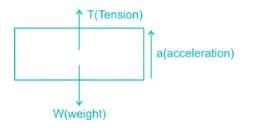Force = T - W

ma = T - W

Acceleration is given as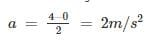Tension is given as

T = mg + ma = m (g + a)

T = 1000 (10 + 2)

T = 12000 N

Engineering Mechanics - 2 - Question 9

A barge is pulled by two tugboats as shown in the figure below. The resultant of forces exerted by the tugboats is 1000 kg force. What will be the value of θ so that tension in rope 2 is minimum?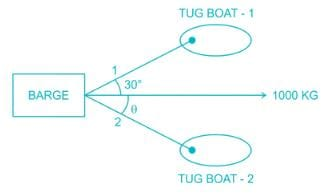Detailed Solution for Engineering Mechanics - 2 - Question 9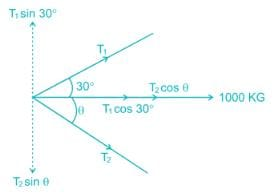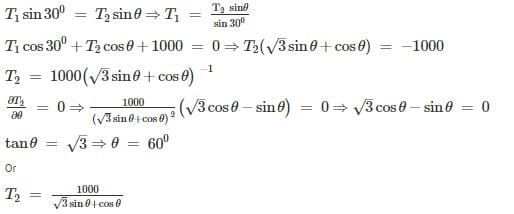By taking the values of θ, given in the options we can conclude that for θ = 600, T2 is minimum.

Engineering Mechanics - 2 - Question 10

The Force applied on a body of mass 100 kg to produce an acceleration of 5 m/s2, is

Detailed Solution for Engineering Mechanics - 2 - Question 10

Acccording to Newton's Second Law of Motion:

F=ma=100×5=500kg−m/s2

Engineering Mechanics - 2 - Question 11

A rubber ball is dropped from a height of 2 m. If there is not loss of velocity after rebounding the ball will rise to a height of

Detailed Solution for Engineering Mechanics - 2 - Question 11

Conservation of energy:

P.E1+K.E1=P.E2+K.E2P.E1+K.E1=P.E2+K.E2

As there is no loss of velocity so

K.E=12mv2⇒K.E1=K.E2K.E=12mv2⇒K.E1=K.E2

P.E1=P.E1⇒h1=h2⇒h2=2m

Engineering Mechanics - 2 - Question 12

A 50 - kg box rests on horizontal floor for which coefficient of friction is 0.3. If the box is subjected to a horizontal towing force of 400 N, what is its velocity after 5 sec from rest? (Take g = 10 m/s2

Detailed Solution for Engineering Mechanics - 2 - Question 12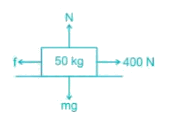Initially checking whether towing force can overcome the static friction force or not.

Friction force (f) = μN = μmg = 0.3 × 50 × 10 = 150 N

∴ Net force on box = 400 – 150 = 250 N

⇒ 250 = ma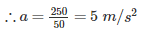Velocity after 5 second

V = u + at = 0 + 5 × 5 = 25 m/s

Engineering Mechanics - 2 - Question 13

A cannonball is fired from a tower 80 m above the ground with a horizontal velocity of 100 m/s. Determine the horizontal distance at which the ball will hit the ground. (Take g = 10 m/s2)

Detailed Solution for Engineering Mechanics - 2 - Question 13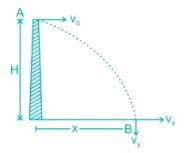vx = vo = Horizontal component of velocity

vy = Vertical component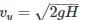Time taken by the body to cover vertical distance H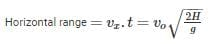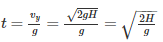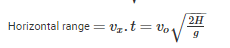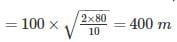Engineering Mechanics - 2 - Question 14

A bucket of water weighing 10 kg is pulled up from a 20 m deep well by a rope weighing 1 kg/m length, then the work done is _____.

Detailed Solution for Engineering Mechanics - 2 - Question 14

Let at a distance of x, weight to be pulled is 10 kg of bucket and x kg of rope weight.

So total weight is (10+x)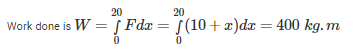Engineering Mechanics - 2 - Question 15

The sum of kinetic and potential energy of a falling body _____.

Detailed Solution for Engineering Mechanics - 2 - Question 15

Kinetic energy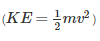represents energy the mass possesses by virtue of its motion. Likewise, potential energy (PE=mgh) represents energy the mass possesses by virtue of its position.

E=KE+PE

E is the total energy of the mass: i.e., the sum of its kinetic and potential energies. It is clear that E is a conserved quantity: i.e., although the kinetic and potential energies of the mass vary as it falls, its total energy remains the same and one form of energy is being converted into other form of energy.

Engineering Mechanics - 2 - Question 16

In case of principal axes of a section _____.

Detailed Solution for Engineering Mechanics - 2 - Question 16

In principal axes of a section product of inertia is zero.

Product of inertia:- The moment of inertia between any two mutually perpendicular axis in the plane of area is the produce of inertia.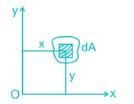Product of inertia,

Ixy=∫dA.xy

Engineering Mechanics - 2 - Question 17

If v1 and v2 are the initial velocities of two bodies making direct collision and if u1 and u2 are their respective velocities after collision then the coefficient of restitution is given by:

Detailed Solution for Engineering Mechanics - 2 - Question 17

The energy dissipation during impact is called by the term, coefficient of restitution, a scalar quantity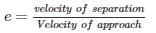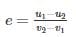Engineering Mechanics - 2 - Question 18

The position of a particle in rectilinear motion is given by the equation (x = t3 - 2t2 + 10t - 4), where x is in meters and t is in seconds. What will be the velocity of the particle at 3s?

Detailed Solution for Engineering Mechanics - 2 - Question 18

Position (x) = t3 – 2t2 + 10t – 4

Velocity (v) =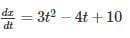At t = 3 s

V = 3 × 32 – 4 × 3 + 10 = 25 m/s

Engineering Mechanics - 2 - Question 19

The angular velocity (in radians /sec) of a body rotating at N rpm is:

Detailed Solution for Engineering Mechanics - 2 - Question 19

Rpm is just a measure of how many times a point is revolving per minute.

In a single revolution, a point rotates an angle = 2π

So, the total angle a point is rotating per minute = N x 2 π

The angular velocity is a measure of how fast it is rotating = Angle Rotated/Time Required = 2π N/60

Engineering Mechanics - 2 - Question 20

For maximum horizontal range, the angle of projection of a projectile should be

Detailed Solution for Engineering Mechanics - 2 - Question 20

Horizontal range,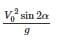For maximum horizontal range.

Sin 2α = 1

Or 2α = 90°

Or α = 45°

## Mechanical Engineering SSC JE (Technical)

6 videos|97 docs|57 tests
Information about Engineering Mechanics - 2 Page
In this test you can find the Exam questions for Engineering Mechanics - 2 solved & explained in the simplest way possible. Besides giving Questions and answers for Engineering Mechanics - 2, EduRev gives you an ample number of Online tests for practice

## Mechanical Engineering SSC JE (Technical)

6 videos|97 docs|57 tests(Scan QR code)